Home

# On the Euler characteristic of spherical polyhedra and the Euler relation

## Extract

Let En+1, for some integer n ≥ 0, be the (n + 1)-dimensional Euclidean space, and denote by Sn the standard n–sphere in En+1,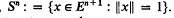. It is convenient to introduce the (–1)-dimensional sphere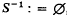, where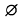denotes the empty set. By an i-dimensional subsphere T of Sn, i = 0 n, we understand the intersection of Sn with some (i+1)-dimensional subspace of En+1. The affine hull of T always contains, with this definition, the origin of En+1.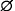is the unique (–1)-dimensional subsphere of Sn. By the spherical hull, sph X, of a set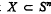, we understand the intersection of all subspheres of Sn containing X. Further we set dim X: = dim sph X. The interior, the boundary and the complement of an arbitrary set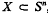, with respect to Sn, shall be denoted by int X, bd X and cpl X. Finally we define the relative interior rel int X to be the interior of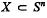with respect to the usual topology sphZ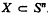. For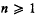each (n–1)-dimensional subsphere of Sn defines two closed hemispheres of Sn, whose common boundary it is. The two hemispheres of the sphere Sº are denned to be the two one-pointed subsets of Sº. A subset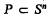is called a closed (spherical) polytope, if it is the intersection of finitely many closed hemispheres, and, if, in addition, it does not contain a subsphere of Sn.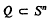is called an i-dimensional, relatively open polytope,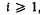, or shortly an i-open polytope, if there exists a closed polytope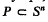such that dim P = i and Q = rel int P.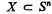is called a closed polyhedron, if it is a finite union of closed polytopes P1 …, Pr. The empty set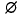is the only (–1)-dimensional closed polyhedron of Sn. We denote by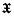the set of all closed polyhedra of Sn.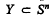is called an i-open polyhedron, for some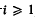, if there are finitely many i-open polytopes Q1 …, Qr in Sn such that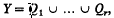, and dim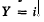. By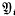we denote the set of all i-open polyhedra. Clearly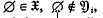for all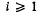, and each i-dimensional subsphere of Sn,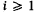, belongs to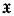and to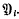, For each i-dimensional subsphere T of Sn, set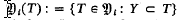. A map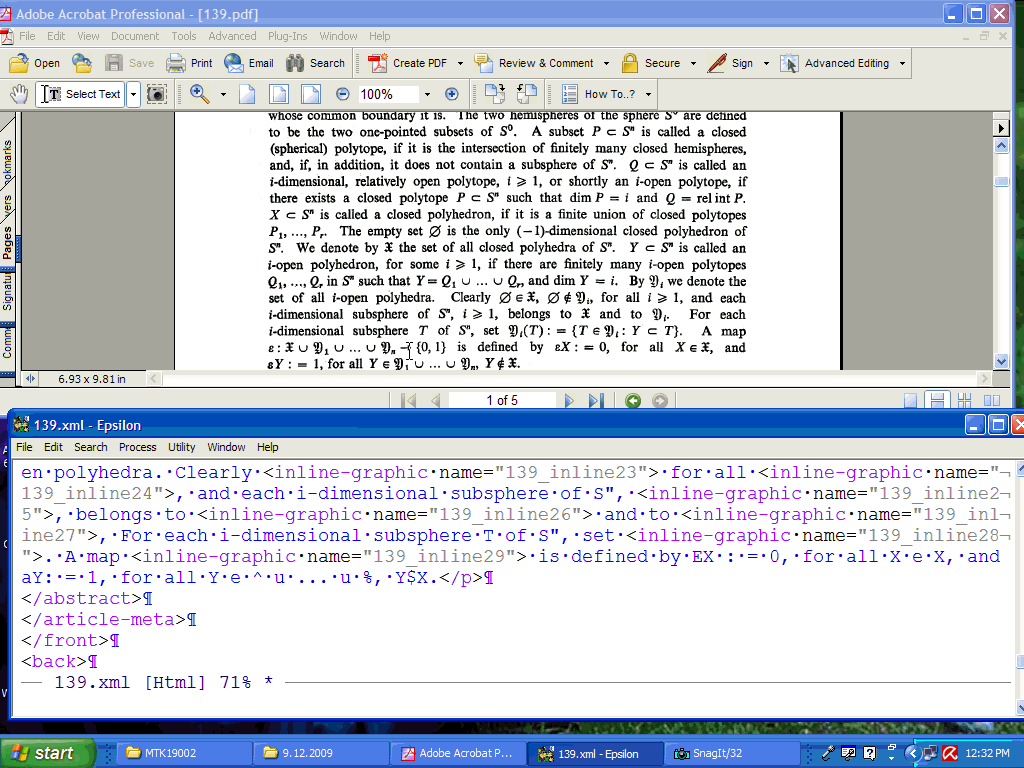is defined by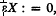, for all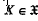, and, for all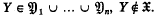.

## References

Hide All
MathJax
MathJax is a JavaScript display engine for mathematics. For more information see http://www.mathjax.org.

# On the Euler characteristic of spherical polyhedra and the Euler relation

## Metrics

### Full text viewsFull text views reflects the number of PDF downloads, PDFs sent to Google Drive, Dropbox and Kindle and HTML full text views.

Total number of HTML views: 0
Total number of PDF views: 0 *Loading metrics...

### Abstract viewsAbstract views reflect the number of visits to the article landing page.

Total abstract views: 0 *Loading metrics...

* Views captured on Cambridge Core between <date>. This data will be updated every 24 hours.

Usage data cannot currently be displayed.